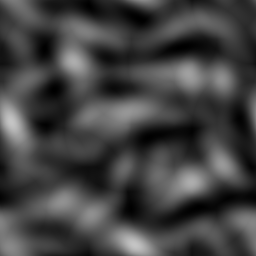# Wavelet noise node¶

The Wavelet noise node outputs a texture generated repetition of a wavelet pattern.## Inputs¶

The Wavelet noise node does not accept any input.

## Outputs¶

The Wavelet noise node provides a greyscale Wavelet noise texture.

## Parameters¶

The Wavelet noise node accepts the following parameters:

• Type defines how octaves are calculated

• Scale X and Scale Y are the subdivisions of the noise

• Iterations is the number of iterations

• Persistence is the strength of each subsequent iteration

• Frequency is the frequency of each wavelet pattern

• Offset is the phase of each wavelet pattern

## Example images¶